# Organic Chemistry Find the Compound Type Questions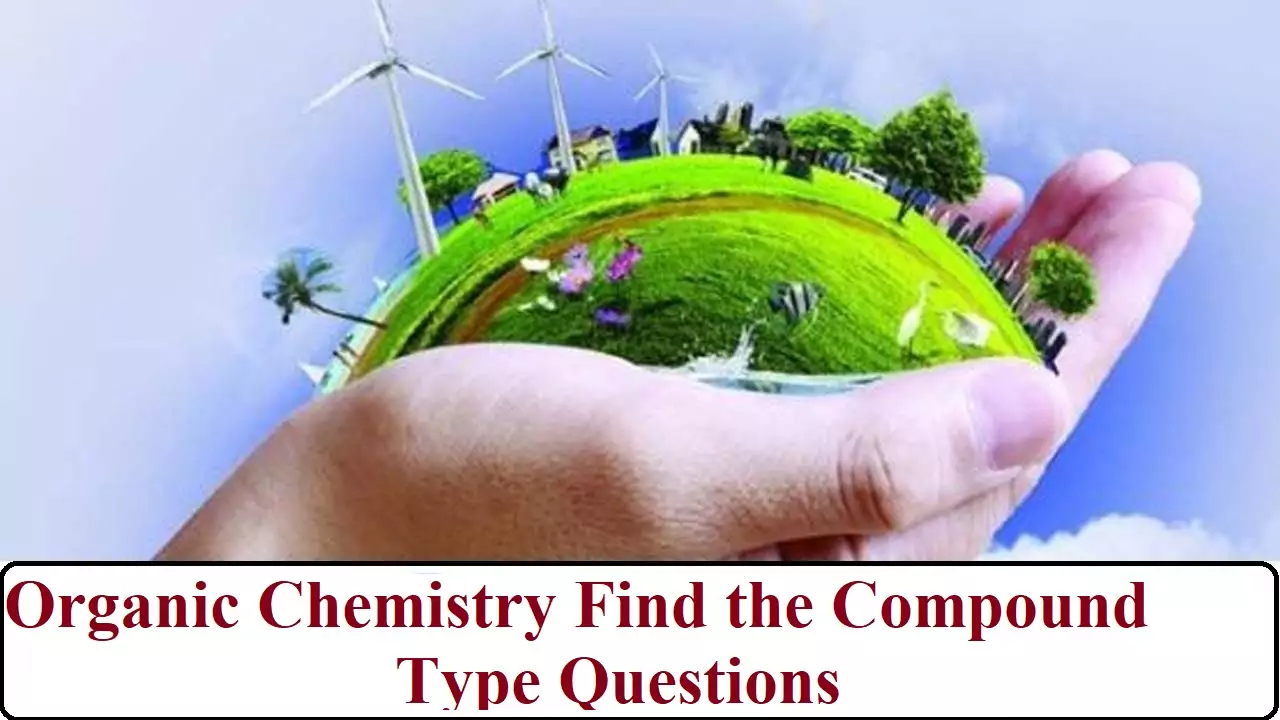Organic Chemistry Find the Compound Type Questions

Que 1. An organic compound (A) (C8H16O2) was hydrolysed with dilute sulphuric acid to give carboxylic acid (B) and alcohol (C). Oxidation of (C) with chromic acid produced (B). (C) on dehydration gives but-1-ene. Identity A,B,C.
Ans 1. Organic compound A is an ester as on acid hydrolysis it gives a mixture of an acid and an alcohol. Oxidation of alcohol (C) gives acid (B). Hence, the number of carbon atoms in (B) and (C) are the same. Ester (compound A) has eight C atoms. Hence, both carboxylic acid B and alcohol C must Contain 4 C atoms each.  Dehydration of alcohol C gives but-1-ene. Hence, C must be straight-chain alcohol, i.e butan-1-ol. Oxidation of (C) gives (B). Hence, (B) is butanoic acid.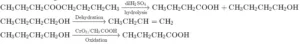Que 2. An organic compound with the molecular formula C9H10O forms 2,4 DNP derivative reduces Tollen’s reagent and undergoes Cannizaro reaction. on vigorous oxidation, it gives 1,2 benzenecarboxylic acid. Identify the compound.
Ans 2. An organic compound with the molecular formula C9​H10​O forms -DNP derivative and also reduces Tollens reagent. Hence, the compound is an aldehyde.
The compound undergoes a Cannizzaro reaction. Hence, it does not contains an alpha H atom. On vigorous oxidation, it gives -benzenedicarboxylic acid. Hence, the -CHO group is directly attached to the benzene ring and the compound is ortho di-substituted benzene.
The compound is -ethylbenzaldehyde.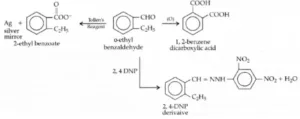Que 3. An organic compound (A) with molecular formula C8H8O forms an orange-red precipitate with 2,4 DNP reagent and gives yellow precipitate on heating with iodine in the presence of sodium hydroxide. It neither reduces Tollen’s or Fehling’s reagent, nor does it decolourise bromine water or Baeyer’s reagents. On drastic oxidation with chromic acid, it gives a carboxylic acid (B) having molecular formula C7H6O2. Identify the compounds (A) and (B).
Ans 3. (A) forms 2,4-DNP derivative. Therefore, it is an aldehyde or a ketone. Since it does not reduce Tollens’ or Fehling reagent, (A) must be a ketone. (A) responds to iodoform test. Therefore, it should be a methyl ketone. The molecular formula of (A) indicates high degree of unsaturation, yet it does not decolourise bromine water or Baeyer’s reagent. This indicates the presence of unsaturation due to an aromatic ring.
Compound (B), being an oxidation product of a ketone should be a carboxylic acid. The molecular formula of (B) indicates that it should be benzoic acid and compound (A) should, therefore, be a monosubstituted aromatic methyl ketone. The molecular formula of (A) indicates that it should be phenyl methyl ketone (acetophenone). Reactions are as follows: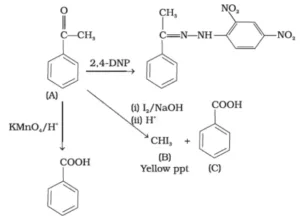Organic Chemistry Find the Compound Type Questions

Que 4. Two moles of organic compound A on treatment with a strong base gives two compounds B and C. Compound B on dehydration with Cu gives A while acidification of C yields carboxylic acid D having a molecular formula of CH2O2. Identify the compounds A, B, C, D.
Ans 4. Since, the molecular formula of D thus, D is HCOOH (formic acid). D is obtained by the acidification of C, so C is sodium formate (HCOONa). Thus, A must be formaldehyde (as it undergoes Cannizzaro reaction with a strong base).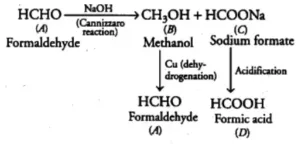Que 5. An aliphatic compound A​’ with a molecular formula of C3H6O reacts with phenylhydrazine to give compound B’. The reaction of A’​ with I2 in alkaline medium on warming gives a yellow precipitate C’​. Identify the component A, B, C.
Ans 5. CH3COCH3 + C6H5NH – NH2 →CH3C = N – HN – C6H5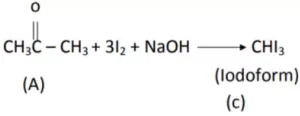Que 6. A component ‘​A’ with molecular formula C5H10O gave a positive 2, 4 DNP test but a negative Tollen’s reagents test. It was oxidised to carboxylic acid B’​ with molecular formula C3H6O2 when treated with alkaline KMnO4 under vigorous conditions. The sodium salt of B​ gave hydrocarbon C’ on Kolbe electrolysis reaction. Identify A, B, C and D.
Ans 6. A) The First compound gives positive DNP test suggests it’s aldehyde/ketone. Negative Tollen’s test indicates its a ketone i.e. pentanone.
Thus, compound A is Pentan-3-one (CH3-CH2-CO-CH2-CH3).
B) Pentan-3-one oxidizes to form propanoic acid.
CH3-CH2-CO-CH2-CH3 + [O] → CH3-CH2-COOH + CH3-COOH
Hence, compound B is propanoic acid (CH3-CH2-COOH).
C) Propanoic acid on Kolbe’s reaction gives n-butane.
CH3-CH2-COONa → CH3-CH2-CH2-CH3
Therefore, Compound C is n-butane (CH3-CH2-CH2-CH3).

Que 7. An organic compound ‘A’​ is resistant to oxidation forms on oxidation a compound ‘B​(C3H8O) on reduction.​‘B’ reacts with HBr to form a bromide C’ which on treatment with alcoholic KOH forms an alkene ‘D’​ (C3H6). Deduce A, B, C, D.
Ans 7.Que 8. Etherial solution of an organic compound A when heated with magnesium gave B. Which on treatment with ethanal followed by acid hydrolysis gave 2-propanol. Identify the compound A. What is ‘B’ known as?
Ans 8.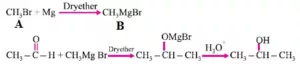Organic Chemistry Find the Compound Type Questions

Que 9. Primary alkyl halide C4H9Br (A) reacted with alcoholic KOH to give compound (B) is reacted with HBr to give (C) which is an isomer of (A). When (A) is reacted with sodium metal it gives compound (D) C8H18 that was different from the compound formed when n-butyl bromide is reacted with sodium. Give the formula of (A) and write equations.
Ans 9. Two primary alkyl halides with molecular formula  are possible. They are n-butyl bromide and isobutyl bromide.
When (a) is reacted with sodium metal it gives compound (d), ​H18 which is different from the compound formed when n-butyl bromide is reacted with sodium. Hence, compound a is isobutyl bromide and compound d is 2, 5-dimethylhexane.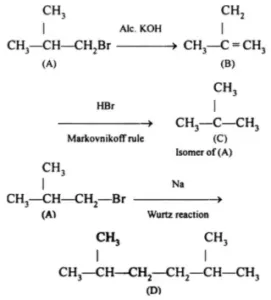Que 10. An ester C6H12O2 was hydrolysed with water an acid (A), and alcohol (B), were obtained. Oxidation of (B) with chromic acid produced A. What is the structure of the original ester? Write equations for all the reactions.
Ans 10.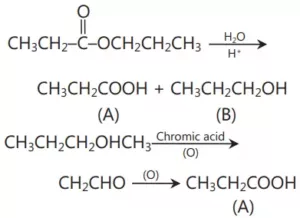Que 11. An unsaturated acid (A) of molecular formula C5H6O4 eliminates CO2 easily and gives another unsaturated acid (B) of formula C4H6O2. By saturation with H2/Pt (B) gives butanoic acid. Neither (A) nor (B) shows cis-trans isomerism. What are (A) and (B)?
Ans 11.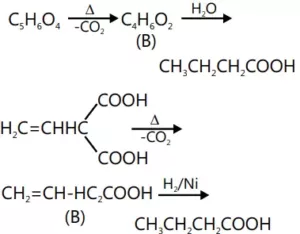Que 12. An organic compound A on treatment with ethyl alcohol gives a carboxylic acid B and compound C. Hydrolysis of C under acidic conditions gives B and D. Oxidation of D with KMnO4 also gives B. The compound B on heating with Ca(OH)2 gives E (molecular formula C3H6O). E does not give Tollen’s test and does not reduce Fehling’s solution but forms a 2,4– dinitrophenylhydrazone. Identify A, B, C, D and E.
Ans 12. The given reactions are as follows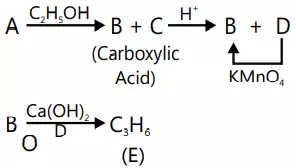The compound E must be ketonic compound as it does not give Tollen’s test and does not reduce Fehling’s solution but forms a 2, 4-dinitrophenyl-hydrazone. Therefore, its structure would be CH3COCH3 (acetone). Since E is obtained by heating B with Ca(OH)2, compound B must be CH3COOH (acetic acid). Since B is obtained by oxidation of D with KMnO4, the compound D must alcohol with molecular formula CH3CH2OH (ethanol). Since B and D are obtained by acid hydrolysis of C, compound C must be an ester CH3COOC2H5 (ethyl acetate). Since the compounds, B (acetic acid) and C (ethyl acetate) are obtained by treating A with ethanol, compound A must be an anhydride (CH3CO)2O (acetic anhydride). The given reactions are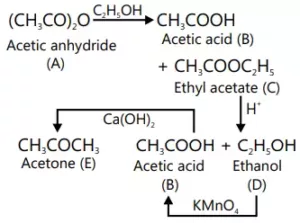Que 13. An organic compound (A) gives positive Liebermann reaction and on treatment with CHCl3/KOH followed by hydrolysis gives (B) and (C). Compound (B) gives colour with Schiff’s reagent but not (C), which is steam volatile. (C) on treatment with LiAIH4 gives (D), C7H8O2, which on oxidation gives (E). Compound (E) reacts with (CH3CO)2O/CH3COOH to give a pain reliever (F). Give the structures of (A) to (F) with proper reasoning.
Ans 13.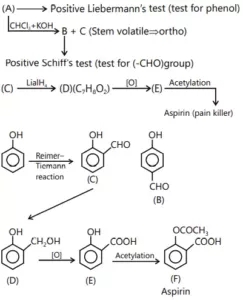Organic Chemistry Find the Compound Type Questions